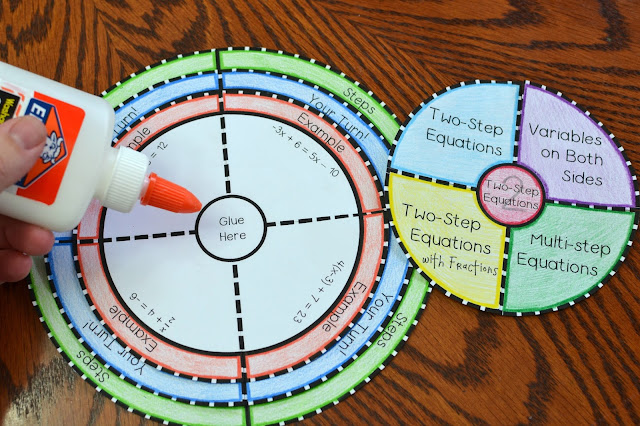### Two Step Equations Foldable

Included is a foldable on two-step equations. This is a great study tool! Students can either use the foldable as a hand-held foldable or glue them into their interactive notebooks.

This foldable has multiple layers. The layer labeled "Examples" is where I use direct instruction to introduce a problem. The layer labeled "Your Turn" is where students will apply what they learned to solve another problem. The layer labeled "Steps" is where students will write down the steps/procedure to solve those type of problems.

Problems include the following:

-Solving two-step equations
-Solving two-step equations involving variables on both sides
-Solving two-step equations with fractions
-Solving multi-step equationsYou can watch my video on this foldable by CLICKING HERE

You can purchase my foldable by CLICKING HERE